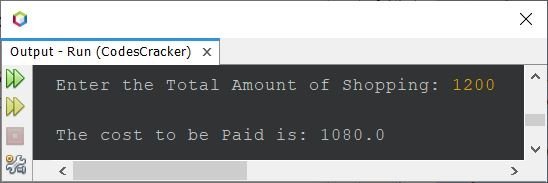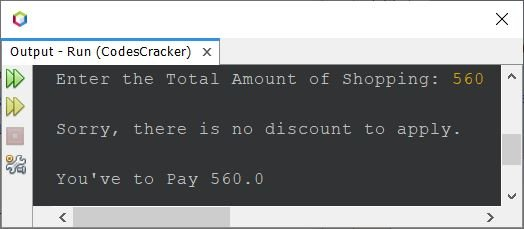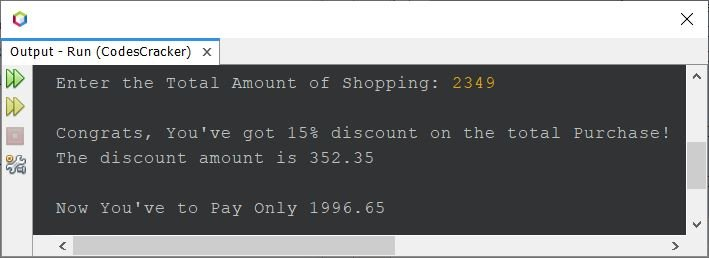# Java Program to Calculate Discount and Price to Paid

This article is created to cover some programs in Java that can calculate the discount and the price that has to be paid after providing the total amount of shopping. The discount must be applied based on the data given in the following table:

Shopping Amount Discount
<=800 No discount
>800 and <=1500 10%
>1500 and <=2500 15%
>2500 and <=5000 20%
>5000 30%

That is, when the total shopping amount is less than \$800, there will be no discount to apply. When the total shopping amount is greater than \$800 and less than or equal to \$1500, then there will be a 10% discount to apply, and so on.

## Discount Program in Java

The question is: write a Java program to find the price that has to be paid after applying the discount (if any). The program given below is the answer to this question:

```import java.util.Scanner;

public class CodesCracker
{
public static void main(String[] args)
{
float totalCost, costToPaid, discount;
Scanner scan = new Scanner(System.in);

System.out.print("Enter the Total Amount of Shopping: ");
totalCost = scan.nextFloat();

if(totalCost<=800)
{
costToPaid = totalCost;
}
else if(totalCost>800 && totalCost<=1500)
{
discount = (totalCost*10)/100;
costToPaid = totalCost - discount;
}
else if(totalCost>1500 && totalCost<=2500)
{
discount = (totalCost*15)/100;
costToPaid = totalCost - discount;
}
else if(totalCost>2500 && totalCost<=5000)
{
discount = (totalCost*20)/100;
costToPaid = totalCost - discount;
}
else
{
discount = (totalCost*30)/100;
costToPaid = totalCost - discount;
}
System.out.println("\nThe cost to be Paid is: " + costToPaid);
}
}```

Here is its sample run with user input 1200 as the total amount of shopping:Since the total amount of shopping is \$1200, after applying the 10% discount, the customer has to pay \$1080 only. But the program looks weird, which means the program is not delivering a good user experience. Therefore, let's modify the above program and recreate it to provide a good user experience:

```import java.util.Scanner;

public class CodesCracker
{
public static void main(String[] args)
{
float totalCost, costToPaid, discount;
Scanner scan = new Scanner(System.in);

System.out.print("Enter the Total Amount of Shopping: ");
totalCost = scan.nextFloat();

if(totalCost<=800)
{
costToPaid = totalCost;
System.out.println("\nSorry, there is no discount to apply.");
}
else if(totalCost>800 && totalCost<=1500)
{
discount = (totalCost*10)/100;
costToPaid = totalCost - discount;
System.out.println("\nCongrats, You've got 10% discount on the total Purchase!");
System.out.println("The discount amount is " +discount);
}
else if(totalCost>1500 && totalCost<=2500)
{
discount = (totalCost*15)/100;
costToPaid = totalCost - discount;
System.out.println("\nCongrats, You've got 15% discount on the total Purchase!");
System.out.println("The discount amount is " +discount);
}
else if(totalCost>2500 && totalCost<=5000)
{
discount = (totalCost*20)/100;
costToPaid = totalCost - discount;
System.out.println("\nCongrats, You've got 20% discount on the total Purchase!");
System.out.println("The discount amount is " +discount);
}
else
{
discount = (totalCost*30)/100;
costToPaid = totalCost - discount;
System.out.println("\nCongrats, You've got 30% discount on the total Purchase!");
System.out.println("The discount amount is " +discount);
}
if(totalCost<=800)
System.out.println("\nYou've to Pay " + costToPaid);
else
System.out.println("\nNow You've to Pay Only " + costToPaid);
}
}```

Here is its sample run with user input 560 as the total purchase amount:Here is another sample run with user input 2349:The program uses if-else statements to do the job. You can re-define the discount based on the shopping range according to your requirements, as this is just a demo program that shows how the discount program using if-else can be created in Java.

Java Online Test

« Previous Program Next Program »